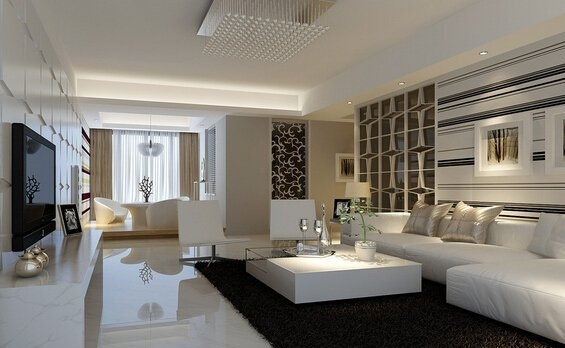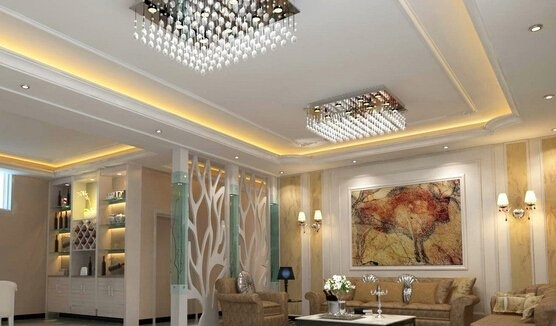|

# 房子装修五大风格详解房子装修五大风格详解

房子装修风格：

一、现代简约风格

简约主义源于20世纪初期的西方现代主义。欧洲现代主义建筑大师Mies、Vander、Rohe的名言“Less、is、more”被认为是代表着简约主义的核心思想。简约主义风格的特色是将设计的元素、色彩、照明、原材料简化到最少的程度，但对色彩、材料的质感要求很高。因此，简约的空间设计通常非常含蓄，往往能达到以少胜多、以简胜繁的效果。

二、传统风格

传统风格的室内设计，是在室内布置、线形、色调以及家具、陈设的造型等方面，吸取传统装饰“形”“神”的特征。例如吸取我国传统木构架建筑室内的藻井天棚、挂落、雀替的构成和装饰，明、清家具造型和款式特征。又如西方传统风格中仿罗马风、哥特式、文艺复兴式、巴洛克、洛可可、古典主义等，其中如仿欧洲英国维多利亚或法国路易式的室内装潢和家具款式。此外，还有日本传统风格、印度传统风格、伊斯兰传统风格、北非城堡风格等等。传统风格常给人们以历史延续和地域文脉的感受，它使室内环境突出了民族文化渊源的形象特征。

三、欧式风格

欧式风格分为几种，其中的巴洛克风格于17世纪盛行欧洲，强调线形流动的变化，色彩华丽。它在形式上以浪漫主义为基础，装修材料常用大理石、多彩的织物、精美的地毯，精致的法国壁挂，整个风格豪华、富丽，充满强烈的动感效果。另一种是洛可可风格，其爱用轻快纤细的曲线装饰，效果典雅、亲切，欧洲的皇宫贵族都偏爱这个风格。

欧式的居室有的不只是豪华大气，更多的是惬意和浪漫。欧式装饰风格最适用于大面积房子，若空间太小，不但无法展现其风格气势，反而对生活在其间的人造成一种压迫感。当然，还要具有一定的美学素养，才能善用欧式风格，否则只会弄巧成拙。房子装修五大风格详解

四、中国古典风格

中国传统的室内设计融合了庄重与优雅双重气质。现在的中式风格更多地利用了后现代手法，把传统的结构形式通过重新设计组合以另一种民族特色的标志符号出现。例如，厅里摆一套明清式的红木家具，墙上挂一幅中国山水画等，传统的书房里自然少不了书柜、书案以及文房四宝。中式的客厅具有内蕴的风格，为了舒服，中式的环境中也常常用到沙发，但颜色仍然体现着中式的古朴，这种表现使整个空间，传统中透着现代，现代中揉着古典。这样就以一种东方人的“留白”美学观念控制的节奏，显出大家风范，其墙壁上的字画无论数量还是内容都不在多，而在于它所营造的意境。可以说无论现在的西风如何劲吹，舒缓的意境始终是东方人特有的情怀，因此书法常常是成就这种诗意的最好手段。这样躺在舒服的沙发上，任千年的故事顺指间流淌。

中国风的构成主要体现在传统家具(多为明清家具为主)、装饰品及黑、红为主的装饰色彩上。室内多采用对称式的布局方式，格调高雅，造型简朴优美，色彩浓重而成熟。中国传统室内陈设包括字画、匾幅、挂屏、盆景、瓷器、古玩、屏风、博古架等，追求一种修身养性的生活境界。中国传统室内装饰艺术的特点是总体布局对称均衡，端正稳健，而在装饰细节上崇尚自然情趣，花鸟、鱼虫等精雕细琢，富于变化，充分体现出中国传统美学精神。

五、地中海风格

通常，“地中海风格”的家居，会采用这么几种设计元素：白灰泥墙、连续的拱廊与拱门，陶砖、海蓝色的屋瓦和门窗。地中海风格的灵魂，目前比较一致的看法就是“蔚蓝色的浪漫情怀，海天一色、艳阳高照的纯美自然”。

地中海风格特征、“地中海风格”的建筑特色是，拱门与半拱门、马蹄状的门窗。建筑中的圆形拱门及回廊通常采用数个连接或以垂直交接的方式，在走动观赏中，出现延伸般的透视感。此外，家中的墙面处(只要不是承重墙)，均可运用半穿凿或者全穿凿的方式来塑造室内的景中窗。这是地中海家居的一个情趣之处。

“地中海风格”对中国城市家居的最大魅力，恐怕来自其纯美的色彩组合。地中海风格有三种典型的颜色搭配。蓝与白、黄、蓝紫和绿、土黄及红褐。

`声明：本文由入驻焦点开放平台的作者撰写，除焦点官方账号外，观点仅代表作者本人，不代表焦点立场错误信息举报电话： 400-099-0099，邮箱：jubao@vip.sohu.com，或点此进行意见反馈，或点此进行举报投诉。`A B C D E F G H J K L M N P Q R S T W X Y Z
A - B - C - D - E
• A
• 鞍山
• 安庆
• 安阳
• 安顺
• 安康
• 澳门
• B
• 北京
• 保定
• 包头
• 巴彦淖尔
• 本溪
• 蚌埠
• 亳州
• 滨州
• 北海
• 百色
• 巴中
• 毕节
• 保山
• 宝鸡
• 白银
• 巴州
• C
• 承德
• 沧州
• 长治
• 赤峰
• 朝阳
• 长春
• 常州
• 滁州
• 池州
• 长沙
• 常德
• 郴州
• 潮州
• 崇左
• 重庆
• 成都
• 楚雄
• 昌都
• 慈溪
• 常熟
• D
• 大同
• 大连
• 丹东
• 大庆
• 东营
• 德州
• 东莞
• 德阳
• 达州
• 大理
• 德宏
• 定西
• 儋州
• 东平
• E
• 鄂尔多斯
• 鄂州
• 恩施
F - G - H - I - J
• F
• 抚顺
• 阜新
• 阜阳
• 福州
• 抚州
• 佛山
• 防城港
• G
• 赣州
• 广州
• 桂林
• 贵港
• 广元
• 广安
• 贵阳
• 固原
• H
• 邯郸
• 衡水
• 呼和浩特
• 呼伦贝尔
• 葫芦岛
• 哈尔滨
• 黑河
• 淮安
• 杭州
• 湖州
• 合肥
• 淮南
• 淮北
• 黄山
• 菏泽
• 鹤壁
• 黄石
• 黄冈
• 衡阳
• 怀化
• 惠州
• 河源
• 贺州
• 河池
• 海口
• 红河
• 汉中
• 海东
• I
• J
• 晋中
• 锦州
• 吉林
• 鸡西
• 佳木斯
• 嘉兴
• 金华
• 景德镇
• 九江
• 吉安
• 济南
• 济宁
• 焦作
• 荆门
• 荆州
• 江门
• 揭阳
• 金昌
• 酒泉
• 嘉峪关
K - L - M - N - P
• K
• 开封
• 昆明
• 昆山
• L
• 廊坊
• 临汾
• 辽阳
• 连云港
• 丽水
• 六安
• 龙岩
• 莱芜
• 临沂
• 聊城
• 洛阳
• 漯河
• 娄底
• 柳州
• 来宾
• 泸州
• 乐山
• 六盘水
• 丽江
• 临沧
• 拉萨
• 林芝
• 兰州
• 陇南
• M
• 牡丹江
• 马鞍山
• 茂名
• 梅州
• 绵阳
• 眉山
• N
• 南京
• 南通
• 宁波
• 南平
• 宁德
• 南昌
• 南阳
• 南宁
• 内江
• 南充
• P
• 盘锦
• 莆田
• 平顶山
• 濮阳
• 攀枝花
• 普洱
• 平凉
Q - R - S - T - W
• Q
• 秦皇岛
• 齐齐哈尔
• 衢州
• 泉州
• 青岛
• 清远
• 钦州
• 黔南
• 曲靖
• 庆阳
• R
• 日照
• 日喀则
• S
• 石家庄
• 沈阳
• 双鸭山
• 绥化
• 上海
• 苏州
• 宿迁
• 绍兴
• 宿州
• 三明
• 上饶
• 三门峡
• 商丘
• 十堰
• 随州
• 邵阳
• 韶关
• 深圳
• 汕头
• 汕尾
• 三亚
• 三沙
• 遂宁
• 山南
• 商洛
• 石嘴山
• T
• 天津
• 唐山
• 太原
• 通辽
• 铁岭
• 泰州
• 台州
• 铜陵
• 泰安
• 铜仁
• 铜川
• 天水
• 天门
• W
• 乌海
• 乌兰察布
• 无锡
• 温州
• 芜湖
• 潍坊
• 威海
• 武汉
• 梧州
• 渭南
• 武威
• 吴忠
• 乌鲁木齐
X - Y - Z
• X
• 邢台
• 徐州
• 宣城
• 厦门
• 新乡
• 许昌
• 信阳
• 襄阳
• 孝感
• 咸宁
• 湘潭
• 湘西
• 西双版纳
• 西安
• 咸阳
• 西宁
• 仙桃
• 西昌
• Y
• 运城
• 营口
• 盐城
• 扬州
• 鹰潭
• 宜春
• 烟台
• 宜昌
• 岳阳
• 益阳
• 永州
• 阳江
• 云浮
• 玉林
• 宜宾
• 雅安
• 玉溪
• 延安
• 榆林
• 银川
• Z
• 张家口
• 镇江
• 舟山
• 漳州
• 淄博
• 枣庄
• 郑州
• 周口
• 驻马店
• 株洲
• 张家界
• 珠海
• 湛江
• 肇庆
• 中山
• 自贡
• 资阳
• 遵义
• 昭通
• 张掖
• 中卫

1室1厅1厨1卫1阳台

1
2
3
4
5

0
1
2

1

1

0
1
2
3报名成功，资料已提交审核A B C D E F G H J K L M N P Q R S T W X Y Z
A - B - C - D - E
• A
• 鞍山
• 安庆
• 安阳
• 安顺
• 安康
• 澳门
• B
• 北京
• 保定
• 包头
• 巴彦淖尔
• 本溪
• 蚌埠
• 亳州
• 滨州
• 北海
• 百色
• 巴中
• 毕节
• 保山
• 宝鸡
• 白银
• 巴州
• C
• 承德
• 沧州
• 长治
• 赤峰
• 朝阳
• 长春
• 常州
• 滁州
• 池州
• 长沙
• 常德
• 郴州
• 潮州
• 崇左
• 重庆
• 成都
• 楚雄
• 昌都
• 慈溪
• 常熟
• D
• 大同
• 大连
• 丹东
• 大庆
• 东营
• 德州
• 东莞
• 德阳
• 达州
• 大理
• 德宏
• 定西
• 儋州
• 东平
• E
• 鄂尔多斯
• 鄂州
• 恩施
F - G - H - I - J
• F
• 抚顺
• 阜新
• 阜阳
• 福州
• 抚州
• 佛山
• 防城港
• G
• 赣州
• 广州
• 桂林
• 贵港
• 广元
• 广安
• 贵阳
• 固原
• H
• 邯郸
• 衡水
• 呼和浩特
• 呼伦贝尔
• 葫芦岛
• 哈尔滨
• 黑河
• 淮安
• 杭州
• 湖州
• 合肥
• 淮南
• 淮北
• 黄山
• 菏泽
• 鹤壁
• 黄石
• 黄冈
• 衡阳
• 怀化
• 惠州
• 河源
• 贺州
• 河池
• 海口
• 红河
• 汉中
• 海东
• I
• J
• 晋中
• 锦州
• 吉林
• 鸡西
• 佳木斯
• 嘉兴
• 金华
• 景德镇
• 九江
• 吉安
• 济南
• 济宁
• 焦作
• 荆门
• 荆州
• 江门
• 揭阳
• 金昌
• 酒泉
• 嘉峪关
K - L - M - N - P
• K
• 开封
• 昆明
• 昆山
• L
• 廊坊
• 临汾
• 辽阳
• 连云港
• 丽水
• 六安
• 龙岩
• 莱芜
• 临沂
• 聊城
• 洛阳
• 漯河
• 娄底
• 柳州
• 来宾
• 泸州
• 乐山
• 六盘水
• 丽江
• 临沧
• 拉萨
• 林芝
• 兰州
• 陇南
• M
• 牡丹江
• 马鞍山
• 茂名
• 梅州
• 绵阳
• 眉山
• N
• 南京
• 南通
• 宁波
• 南平
• 宁德
• 南昌
• 南阳
• 南宁
• 内江
• 南充
• P
• 盘锦
• 莆田
• 平顶山
• 濮阳
• 攀枝花
• 普洱
• 平凉
Q - R - S - T - W
• Q
• 秦皇岛
• 齐齐哈尔
• 衢州
• 泉州
• 青岛
• 清远
• 钦州
• 黔南
• 曲靖
• 庆阳
• R
• 日照
• 日喀则
• S
• 石家庄
• 沈阳
• 双鸭山
• 绥化
• 上海
• 苏州
• 宿迁
• 绍兴
• 宿州
• 三明
• 上饶
• 三门峡
• 商丘
• 十堰
• 随州
• 邵阳
• 韶关
• 深圳
• 汕头
• 汕尾
• 三亚
• 三沙
• 遂宁
• 山南
• 商洛
• 石嘴山
• T
• 天津
• 唐山
• 太原
• 通辽
• 铁岭
• 泰州
• 台州
• 铜陵
• 泰安
• 铜仁
• 铜川
• 天水
• 天门
• W
• 乌海
• 乌兰察布
• 无锡
• 温州
• 芜湖
• 潍坊
• 威海
• 武汉
• 梧州
• 渭南
• 武威
• 吴忠
• 乌鲁木齐
X - Y - Z
• X
• 邢台
• 徐州
• 宣城
• 厦门
• 新乡
• 许昌
• 信阳
• 襄阳
• 孝感
• 咸宁
• 湘潭
• 湘西
• 西双版纳
• 西安
• 咸阳
• 西宁
• 仙桃
• 西昌
• Y
• 运城
• 营口
• 盐城
• 扬州
• 鹰潭
• 宜春
• 烟台
• 宜昌
• 岳阳
• 益阳
• 永州
• 阳江
• 云浮
• 玉林
• 宜宾
• 雅安
• 玉溪
• 延安
• 榆林
• 银川
• Z
• 张家口
• 镇江
• 舟山
• 漳州
• 淄博
• 枣庄
• 郑州
• 周口
• 驻马店
• 株洲
• 张家界
• 珠海
• 湛江
• 肇庆
• 中山
• 自贡
• 资阳
• 遵义
• 昭通
• 张掖
• 中卫• 手机• 分享
• 设计
免费设计
• 计算器
装修计算器
• 入驻
合作入驻
• 联系
联系我们
• 置顶
返回顶部